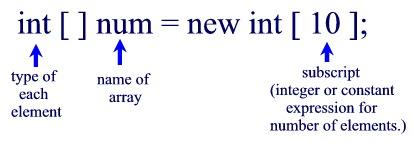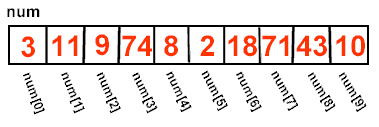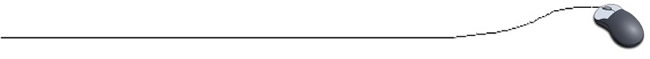Declaring Arrays
Let's declare an array of 10 integer values.This declaration declares an array named num that contains 10 integers.  When the compiler encounters this declaration, it immediately sets aside enough memory to hold all 10 elements.

The square brackets ([ ]) after the "type" indicate that
num is going to be an array of type int rather than a single instance of an int Since the new operator creates (defines) the array, it must know the type and size of the array.  The new operator locates a block of memory large enough to contain the array and associates the array name, num, with this memory block.A program can access each of the array elements (the individual cells) by referring to the name of the array followed by the subscript denoting the element (cell).  For example, the third element is denoted num.Mantra: The name of the array is the address of the first element of the array.

The subscripts of array elements begin with zero.

The first subscript is always zero and the last subscript's value is
(length - 1), where length designates the number of elements within the array
(which is set when the array is declared).

Consider the following possible (?) subscripts for our array:
int [ ] num = new int ;

 num [ 0 ] always OK num [ 9 ] OK  (given the above declaration) num [ 10 ] illegal (no such cell from this declaration) num [ -1 ] always NO! (illegal) num [ 3.5 ] always NO! (illegal)

If the value of an index for an array element is negative, a decimal, or greater than or equal to the length of the array (remember that the last subscript is array length - 1),
an error message will be ArrayIndexOutOfBoundsException.
If you see this message, immediately check to see how your array is being utilized.

Array Length:  It is possible that the length of an array may be unknown or that it may change based upon the source of the data used. When dealing with arrays, it is advantageous to know the number of elements contained within the array, or the array's "length".  This length can be obtained by using the array name followed by .length.  If an array named numbers contains 10 values, the code numbers.length will be 10.  ** You must remember that the length of an array is the number of elements in the array, which is one more than the largest subscript. The subscripts of arrays start with zero.value.length is a property used to find the length of an array named value. value.length( ) is a method used to find the length of a String named value (not an array)

Remember: when finding the length of an array, do NOT include parentheses after length.

 We will be using the syntax shown above for declaring and instantiating arrays. There are, however, several different syntax options available in Java. The following three options are all acceptable: // one statement int [ ] num = new int ; // two separate statements int [ ] num; // declare num = new int ; // instantiate // brackets after variable name int num [ ] = new int [ 10];